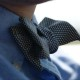# Estimation with the QAMA calculator

I first heard about the QAMA calculator a few weeks ago, and was immediately intrigued.  The QAMA website advertises its device as

The revolutionary calculator that shows the answer only when you also enter a suitable mental estimate.

That’s a good enough hook for me, so 5 devices were ordered, and I had the first chance to work with a group of students using the QAMA calc.  Students in a 7th grade class rotated through learning stations, where working with me on “percentage of a number” problems were a station challenge.

To start, I had students enter the problem 2.8 x 4.9.  Pressing the equals key, students were not given the answer, and instead must give an estimate of the answer.  An answer deemed “reasonable” will then produce the actual answer.  Here, the students agreed that 3 x 5 = 15 would be a reasonable estimate.

From this introduction, we dove into the first percent problem:  what is 78% of 210.  After writing the problem as a decimal multiplication problem, we brainstormed estimation ideas:  75% is close to 78%, and 200 is pretty close to 210 as well.  This led to discussion on parts of 200:  what is 25%, what is half, how much is 75%.  An estimate of 150 was deemed close enough, and the students were hooked.  Students worked at their own pace through the problems, and were excited when their estimate was considered close enough.One of the trickier problems, and one which caused the most discussion, was 8% of 45.  After agreeing that .08, rather than .80, was needed here, honing in on an estimate was a tough ride.  Can we find 10% of 45?  How much less do we need to shave off?  The calculator apparently adjusts its tolerance based on the sophistication of the problem, so some close answers were not allowed.

This problem also yielded the strangest accepted estimate of the day:If anyone can figure out the logic here, I’d be interested to hear it.  Insight into the complexity of the estimation algorithm can be found on the company’s website.  EDIT:  as the folks at QAMA explained to me, the calculator will simply give you the correct answer after 5 incorrect guesses.  This particular student was all over the map with his guesses, so I would not be surprised if this photo represents his 5th guess.

Also, one feature I like is that you can shut off the estimation feature, but the calculator has flashing red lights to let the teacher know the feature was disabled.  Pretty sneaky!

But, this was a fruitful activity, which allowed students to communicate their number sense, and verify their estimates.  Looking forward to hearing more stories of the QAMA calculator.

### 4 responses to “Estimation with the QAMA calculator”

1.mrcbaker4math

You are the first person I have heard from that had an actual classroom experience with them. I am more open to them now – if you were to ask me out of context of QAMA, I would tell you that I highly value number sense. Here’s my question – do you see these more as a stations-type tool, or an everyday calculator for a middle/high school student?

•Bob Lochel

Tough call. My curriculum director bought one for his son, as he understands the value of establishing number sense. They are \$20 each, so we’d have to weigh the value of buying class sets. Ideally, I feel they should be used every day, so that the estimation becomes a part of the problem-solving culture. A station activity now and then would probably not be enough.

•mrcbaker4math

These are my issues with them as an everyday calculator:
1. We’d have to choose these over more class set graphing calculators
2. For students (families) buying their own, I see this as a choice between a QAMA or a graphing calc, and I think in the long run there is way more upside to the graphing calc

Another question – if I was going to select a few students to go hard with QAMAs, do you see it an an enrichment tool, or one for intervention?

•Bob Lochel

Definitely as an intervention tool, as opposed to enrichment. The conversations I had with students in the station revealed some of their misunderstanding about number, and some students took pride in sharing their estimates. I can still have activities to support number sense with enrichment students, but would not need the device for it.